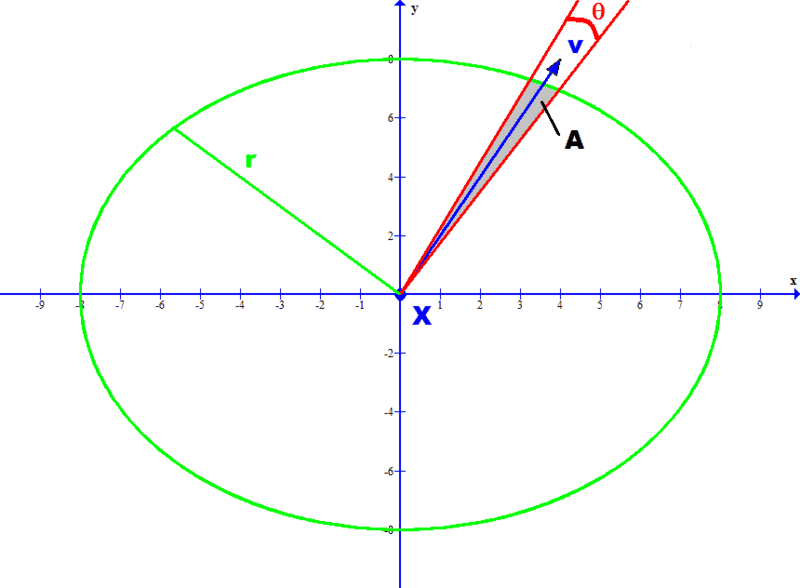# Angular sector

• I
"Consider now an angular sector A(x, r, θ, v) inside a small disk B(x, r) of small angular aperture θ (measured in radians) centred around some direction v (a unit vector) emanating from x; this sector is well-defined, basically because the Riemannian metric gives us the notions of distance and angle."

I have googled and not found any reference to angular sector but have found references to circular sector. is there a difference and if there is what is an angular sector?

fresh_42
Mentor
I assume he says angular instead of circular, as the underlying geometry isn't necessarily Euclidean. It has also the advantage to denote it by an ##A## as in area, whereas a ##C## is occupied by too many other meanings. But the main difference is probably, because ##A(x,r,\theta,v)## isn't flat. One could now object that the ball isn't either, but this would lead too far. In the end it's some sector on a curved surface.

Thanks. So what is the formula for calculating A using the parameters referenced? A picture or illustration would be great so I can see what exactly the variables refer to.

fresh_42
MentorNow you just have to imagine, that ##A(x,r,\theta,v)## isn't part of a flat plane but part of a somehow curved surface.